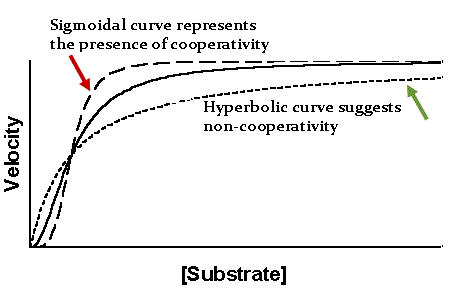# Sigmoid Kinetics

$$\newcommand{\vecs}{\overset { \rightharpoonup} {\mathbf{#1}} }$$ $$\newcommand{\vecd}{\overset{-\!-\!\rightharpoonup}{\vphantom{a}\smash {#1}}}$$$$\newcommand{\id}{\mathrm{id}}$$ $$\newcommand{\Span}{\mathrm{span}}$$ $$\newcommand{\kernel}{\mathrm{null}\,}$$ $$\newcommand{\range}{\mathrm{range}\,}$$ $$\newcommand{\RealPart}{\mathrm{Re}}$$ $$\newcommand{\ImaginaryPart}{\mathrm{Im}}$$ $$\newcommand{\Argument}{\mathrm{Arg}}$$ $$\newcommand{\norm}{\| #1 \|}$$ $$\newcommand{\inner}{\langle #1, #2 \rangle}$$ $$\newcommand{\Span}{\mathrm{span}}$$ $$\newcommand{\id}{\mathrm{id}}$$ $$\newcommand{\Span}{\mathrm{span}}$$ $$\newcommand{\kernel}{\mathrm{null}\,}$$ $$\newcommand{\range}{\mathrm{range}\,}$$ $$\newcommand{\RealPart}{\mathrm{Re}}$$ $$\newcommand{\ImaginaryPart}{\mathrm{Im}}$$ $$\newcommand{\Argument}{\mathrm{Arg}}$$ $$\newcommand{\norm}{\| #1 \|}$$ $$\newcommand{\inner}{\langle #1, #2 \rangle}$$ $$\newcommand{\Span}{\mathrm{span}}$$$$\newcommand{\AA}{\unicode[.8,0]{x212B}}$$

Sigmoidal kinetic profiles are the result of enzymes that demonstrate positive cooperative binding. cooperativity refers to the observation that binding of the substrate or ligand at one binding site affects the affinity of other sites for their substrates. For enzymatic reactions with multiple substrate binding sites, this increased affinity for the substrate causes a rapid and coordinated increase in the velocity of the reaction at higher $$[S]$$ until $$V_{max}$$ is achieved. Plotting the $$V_0$$ vs. $$[S]$$ for a cooperative enzyme, we observe the characteristic sigmoidal shape with low enzyme activity at low substrate concentration and a rapid and immediate increase in enzyme activity to $$V_{max}$$ as $$[S]$$ increases. The phenomenon of cooperativity was initially observed in the oxygen-hemoglobin interaction that functions in carrying oxygen in blood. Positive cooperativity implies allosteric binding – binding of the ligand at one site increases the enzyme’s affinity for another ligand at a site different from the other site. Enzymes that demonstrate cooperativity are defined as allosteric. There are several types of allosteric interactions: (positive & negative) homotropic and heterotropic

.Figure 1: Rate of Reaction (velocity) vs. Substrate Concentration.

Positive and negative allosteric interactions (as illustrated through the phenomenon of cooperativity) refer to the enzyme's binding affinity for other ligands at other sites, as a result of ligand binding at the initial binding site. When the ligands interacting are all the same compounds, the effect of the allosteric interaction is considered homotropic. When the ligands interacting are different, the effect of the allosteric interaction is considered heterotropic. It is also very important to remember that allosteric interactions tend to be driven by ATP hydrolysis.

## Hill Coefficient

The degree of cooperativity is determined by Hill equation (Equation 1) for non-Michaelis-Menten kinetics. The Hill equation accounts for allosteric binding at sites other than the active site. $$n$$ is the "Hill coefficient." When n < 1, there is negative cooperativity; When n = 1, there is no cooperativity; When $$n > 1$$, there is positive cooperativity

$\theta = \dfrac{[L]^n}{K_d+[L]^n} = \dfrac{[L]^n}{K_a^n+[L]^n} \label{1}$

where

• $$\theta$$ is the fraction of ligand binding sites filled
• $$[L]$$ is the ligand concentration
• $$K_d$$ is the apparent dissociation constant derived from the law of mass action (equilibrium constant for dissociation)
• $$K_a$$ is the ligand concentration producing half occupation (ligand concentration occupying half of the binding sides), that is also the microscopic dissociation constant
• $$n$$ is the Hill coefficient that describes the cooperativity

Taking the logarithm of both sides of the equation leads to an alternative formulation of the HIll Equation.

$\log \left( \dfrac{\theta}{1-\theta} \right) = n\log [L] - \log K_d \label{2}$

Currently, there are 2 models for illustrating cooperativity: the concerted model and the sequential model

• The concerted model illustrates cooperativity by assuming that proteins have two or more subunits, and that each part of the protein molecule is able to exist in either the relaxed (R) state or the tense (T) state - the tense state of a protein molecule is favored when it doesn't have any substrates bound. All aspects, including binding and dissociation constants are the same for each ligand at the respective binding sites. This model can also be referred to as the Monod-Wyman-Changeux model, as named after its founders.
• The sequential model aims to demonstrate cooperativity by assuming that the enzyme/protein molecule affinity is relative and changes as substrates bind. Unlike the concerted model, the sequential model accounts for different species of the protein molecule.

## References

1. Raymond Chang. Physical Chemistry for the biosciences. University Science Books. 2005
2. Biological Sciences Review Notes. Kaplan, Inc. 2007
3. "The Hill equation revisited: uses and misuses." J N Weiss